# Pearson's correlation using Minitab

## Introduction

The Pearson product-moment correlation, often shortened to Pearson correlation or Pearson's correlation, is used to assess the strength and direction of association between two continuous variables that are linearly related. Its coefficient, r, indicates the strength and direction of this relationship and can range from -1 for a perfect negative linear relationship to +1 for a perfect positive linear relationship. A value of 0 (zero) indicates that there is no relationship between the two variables.

For example, you could use a Pearson's correlation to understand whether there is an association between test performance and revision time (i.e., your two variables would be "test performance", measured as the exam mark achieved, and "revision time", measured in hours per week). If there was a strong, positive association, we could say that more time spent revising was associated with higher test performance. Alternately, you could use a Pearson's correlation to understand whether there is an association between blood pressure and time spent exercising (i.e., your two variables would be "blood pressure", measured in mm/Hg, and "time spent exercising", measured in hours per week). If there was a moderate, negative association, we could say that exercising more per week is associated with lower blood pressure.

In this guide, we show you how to carry out a Pearson's correlation using Minitab, as well as interpret and report the results from this test. However, before we introduce you to this procedure, you need to understand the different assumptions that your data must meet in order for a Pearson's correlation to give you a valid result. We discuss these assumptions next.

## Assumptions

A Pearson's correlation has four assumptions. You cannot test the first of these assumptions with Minitab because it relates to your study design and choice of variables. However, you should check whether your study meets this assumption before moving on. If this assumption is not met, there is likely to be a different statistical test that you can use instead. Assumption #1 is explained below:

• Assumption #1: Your two variables should be measured at the continuous level (i.e., they are interval or ratio variables). Examples of such continuous variables include height (measured in feet and inches), temperature (measured in °C), salary (measured in US dollars), revision time (measured in hours), intelligence (measured using IQ score), firm size (measured in terms of the number of employees), age (measured in years), reaction time (measured in milliseconds), grip strength (measured in kg), power output (measured in watts), test performance (measured from 0 to 100), sales (measured in number of transactions per month), academic achievement (measured in terms of GMAT score), and so forth. If you are unsure whether your dependent variable is continuous (i.e., measured at the interval or ratio level), see our Types of Variable guide.
• Note: If either of your two variables were measured on an ordinal scale, you need to use Spearman's correlation instead of Pearson's correlation. Examples of ordinal variables include Likert scales (e.g., a 7-point scale from "strongly agree" through to "strongly disagree"), amongst other ways of ranking categories (e.g., a 5-point scale for measuring job satisfaction, ranging from "most satisfied" to "least satisfied"; or a 3-point scale explaining how much a customer liked a product, ranging from "Not very much" to "Yes, a lot").

Assumptions #2, #3 and #4 relate to the nature of your data and can be checked using Minitab. You have to check that your data meets these assumptions because if it does not, the results you get when running a Pearson's correlation might not be valid. In fact, do not be surprised if your data violates one or more of these assumptions. This is not uncommon. However, there are possible solutions to correct such violations (e.g., transforming your data) such that you can still use a Pearson's correlation. Assumptions #2, #3 and #4 are explained below:

• Assumption #2: There needs to be a linear relationship between your two variables. Whilst there are a number of ways to check whether a linear relationship exists, we suggest creating a scatterplot using Minitab, where you can plot your two variables against each other. You can then visually inspect the scatterplot to check for linearity. If the relationship displayed in your scatterplot is not linear, you will have to either "transform" your data or run a Spearman's correlation instead, which you can do using Minitab.
• Assumption #3: There should be no significant outliers. An outlier is simply a case within your data set that does not follow the usual pattern. For example, consider a study examining the relationship between test anxiety of 500 students (where anxiety was measured on a scale of 0-100, with 0 = no anxiety and 100 = maximum anxiety) and exam performance (on a scale from 0 to 100, with 100 the top score). If most participants that had an anxiety score of around 70 had an exam score of around 45, a participant with an anxiety score of 70 who scored 90 in the exam (i.e., an unusually high score) might be an outlier. Pearson's r is sensitive to outliers, which can have a very large effect on the line of best fit and the Pearson correlation coefficient, leading to very difficult conclusions regarding your data. Therefore, it is best if there are no outliers or they are kept to a minimum. Fortunately, you can create scatterplots in Minitab to detect possible outliers.
• Assumption #4: Your variables should be approximately normally distributed. In order to assess the statistical significance of the Pearson correlation, you need to have bivariate normality, but this assumption is difficult to assess, so the simpler method of assessing the normality of each variable separately is more commonly used. This can be done using the Shapiro-Wilk test for normality, which you can carry out using Minitab.

In practice, checking for assumptions #2, #3 and #4 will probably take up most of your time when carrying out a Pearson's correlation. However, it is not a difficult task and Minitab provides all the tools you need to do this.

In the section, Test Procedure in Minitab, we illustrate the Minitab procedure required to perform a Pearson's correlation assuming that no assumptions have been violated. First, we set out the example we use to explain the Pearson's correlation procedure in Minitab.

## Example

An educator wants to determine whether students' exam scores were related to revision time. For example, as students spent more time revising, did their exam score also increase (i.e., a positive relationship) or did the opposite happen (i.e., a negative relationship)? As such, scores in a maths exam were measured on a scale from 0 to 100 and the amount of time spent revising was measured in hours.

To carry out the analysis, the researcher recruited 40 students. The amount of time revising (i.e., the variable, Revision time) and the exam scores (i.e., the variable, Exam score) were recorded for all 40 participants. Expressed in variable terms, the researcher wanted to correlate Exam score and Revision time. A Pearson's correlation was used to determine whether there was a statistically significant relationship between exam score and revision time.

Note: The example and data used for this guide are fictitious. We have just created them for the purposes of this guide.

## Setup in Minitab

In Minitab, we entered our two variables into the first two columns (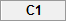and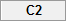). Under columnwe entered the name of one of the two variables, Exam score, as follows: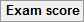. Then, under columnwe entered the name of the second of our two variables, Revision time, as follows: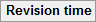. Finally, we entered the scores for Exam score into thecolumn and the scores for Revision time into thecolumn. This is illustrated below: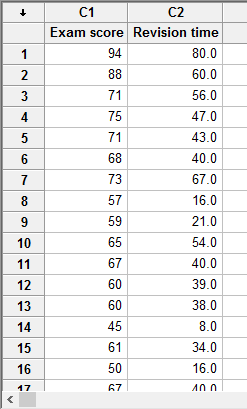Published with written permission from Minitab Inc.

Note: It does not matter which of your two variables you enter under C1 or C2. We have just entered the data into Minitab this way in our example.

## Test Procedure in Minitab

In this section, we show you how to analyse your data using a Pearson's correlation in Minitab when the four assumptions in the previous section, Assumptions, have not been violated. Therefore, the three steps required to run a Pearson's correlation in Minitab are shown below:

• Click Stat > Basic Statistics > Correlation... on the top menu, as shown below: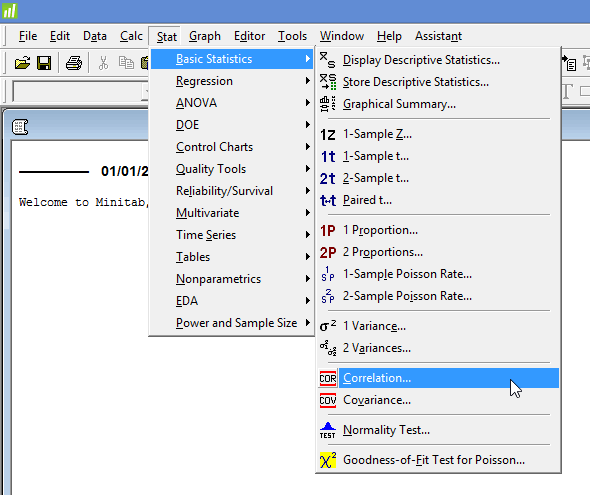Published with written permission from Minitab Inc.

You will be presented with the following Correlation dialogue box: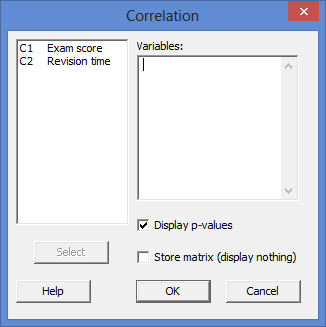Published with written permission from Minitab Inc.

Note: In Minitab 17 you will be presented with only a slightly different dialogue box, as shown below: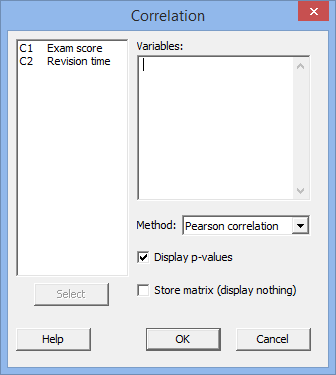If you are using Minitab 17 you need to make sure that "Pearson correlation" is selected as the Method: option (i.e.,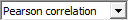).

• Transfer your two variables, Exam score and Revision time from the main left-hand box (i.e., C1   Exam score and C2   Revision time) into the Variables: box. You will end up with the dialogue box shown below: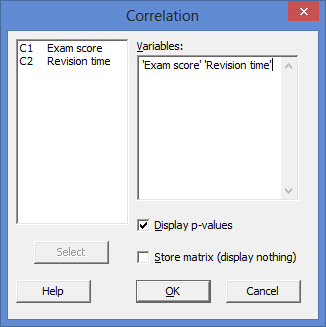Published with written permission from Minitab Inc.

Note: To transfer the two variables, you first need to click inside the main left-hand box and select both variables (e.g., C1   Exam score and C2   Revision time). This will activate the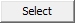button (it is usually faded: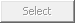). Then, simply press thebutton. Alternately, you can simply double-click on the two variables (i.e., C1   Exam score and C2   Revision time), which will automatically transfer them into the Variables: box.

• Click the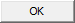button. The output that Minitab produces is shown below.

## Output of the Pearson's correlation in Minitab

The Minitab output for a Pearson's correlation is shown below: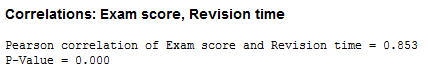The output contains two important pieces of information:

• A. Pearson's correlation coefficient, r. This assesses the strength of the association between the two variables (i.e., Exam score and Revision Time).
• B. The two-tailed statistical significance of Pearson's correlation coefficient (i.e., the p-value).

In this example, Pearson's correlation coefficient is 0.853 (i.e., the "Pearson correlation of Exam score and Revision Time = 0.853" line), which indicates a strong positive correlation between the two variables, Exam score and Revision time, according to Cohen (1998):

 Coefficient Value Strength of Association 0.1 < | r | < .3 small correlation 0.3 < | r | < .5 medium/moderate correlation | r | > .5 large/strong correlation

where | r | means the absolute value or r (e.g., | r | > .5 means r > .5 and r < -.5). Therefore, the Pearson correlation coefficient in this example (r = .853) suggests a strong correlation. If instead, r = -.853, you would also have had a strong correlation, albeit a negative one. This result shows that more time spent revising is associated with better (i.e., higher) exam scores. You can also see that Pearson's correlation coefficient is statistically significant as p < .0005 (i.e., the "P-Value = 0.000" line, which actually means p < .0005), which satisfies the common cut-off for statistical significance of p < .05.

Note: We present the output from the Pearson's correlation above. However, since you should have tested your data for the assumptions we explained earlier in the Assumptions section, you will also need to interpret the Minitab output that was produced when you tested for these assumptions. This includes: (a) the scatterplots you used to check if there was a linear relationship between your two variables (i.e., Assumption #2); (b) the same scatterplots that you will have used to check there were no significant outliers (i.e., Assumption #3); and (c) the Shapiro-Wilk test for normality to check whether your two variables were approximately normally distributed (i.e., Assumption #4). Also, remember that if your data failed any of these assumptions, the output that you get from the Pearson's correlation procedure (i.e., the output we discuss above) might no longer be valid, and you may have to carry out a different statistical test to analyse your data.

## Reporting the output of a Pearson's correlation

When you report the output of your Pearson's correlation, it is good practice to include:

• A. An introduction to the analysis you carried out.